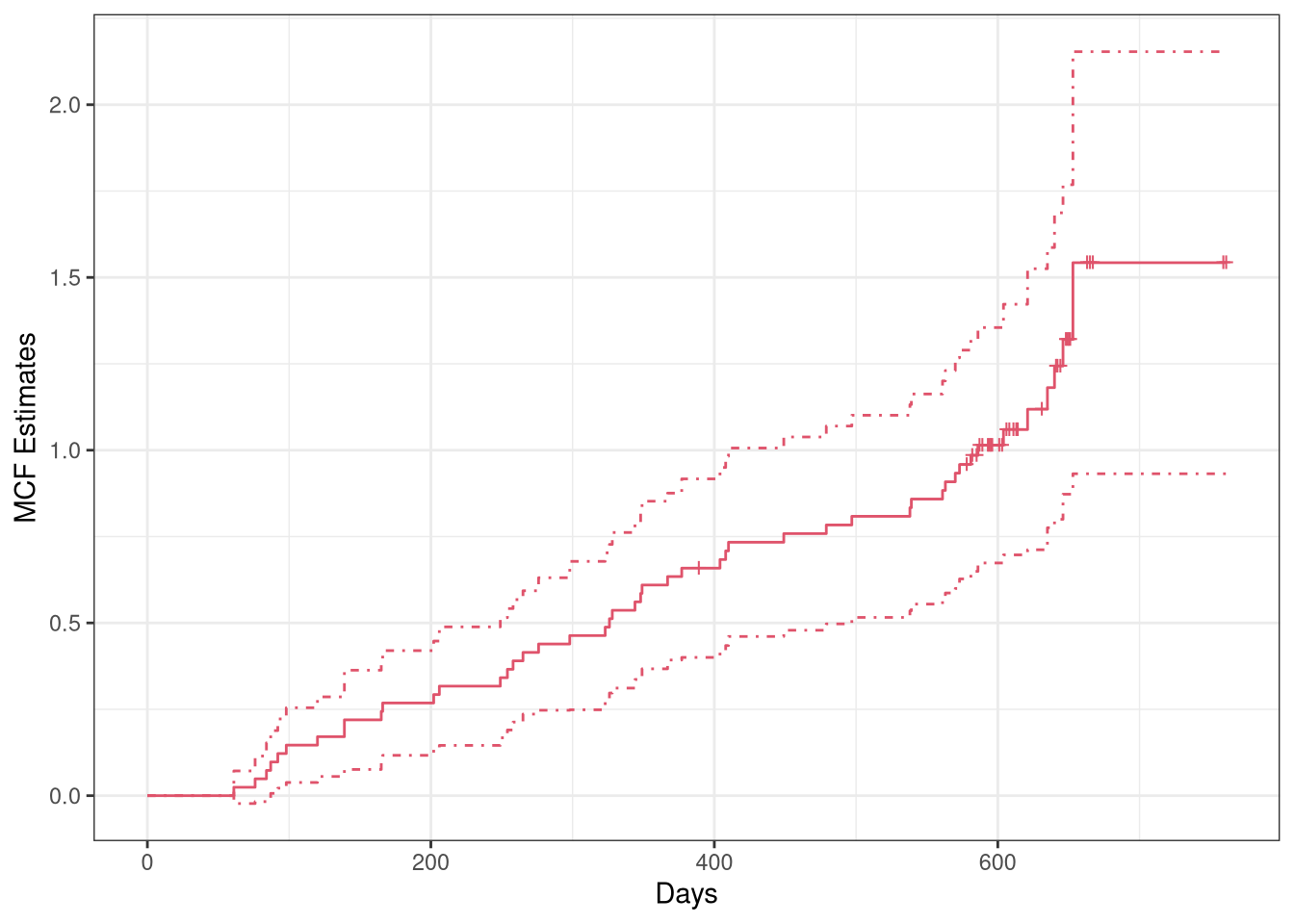In this vignette, we introduce how to explore recurrent event data by mean cumulative function, and modeling the event counts of interest by gamma frailty model with the reda package through examples. Most functions in the package are S4 methods that produce S4 class objects. The details of function syntax and the produced objects are available in the package manual, which will thus not be covered in this vignette.

# Introduction

## Simulated recurrent event data

library(reda)
packageVersion("reda")
##  '0.5.2'

First of all, the sample recurrent event data we are going to use in the following examples is called simuDat, which contains totally 500 observations of 6 variables.

head(simuDat)
##   ID time event group    x1 gender
## 1  1    1     1 Contr -1.93 female
## 2  1   22     1 Contr -1.93 female
## 3  1   23     1 Contr -1.93 female
## 4  1   57     1 Contr -1.93 female
## 5  1  112     0 Contr -1.93 female
## 6  2  140     0 Treat -0.11 female
str(simuDat)
## 'data.frame':    500 obs. of  6 variables:
##  $ID : num 1 1 1 1 1 2 3 3 4 4 ... ##$ time  : num  1 22 23 57 112 140 40 168 14 112 ...
##  $event : int 1 1 1 1 0 0 1 0 1 0 ... ##$ group : Factor w/ 2 levels "Contr","Treat": 1 1 1 1 1 2 1 1 1 1 ...
##  $x1 : num -1.93 -1.93 -1.93 -1.93 -1.93 -0.11 0.2 0.2 -0.43 -0.43 ... ##$ gender: Factor w/ 2 levels "female","male": 1 1 1 1 1 1 1 1 1 1 ...

where

• ID: Subjects identification (ID).
• time: Event or censoring time.
• event: Event indicator, 1 = event; 0 = censored.
• group: Treatment group indicator.
• x1: Continuous variable.
• gender: Gender of subjects.

The dataset was simulated by thinning method (Lewis and Shedler 1979) and further processed for a better demonstration purpose. (Note that reda also provides functions for simulating survival data, and recurrent event data. See vignette("reda-simulate") for details.)

## Data checking

The process’s ID, event times, event indicators or costs, time origins, and possible terminal events of the follow-up is specified in the function Recur(), which serves as the formula response and contains considerate data checking procedures for recurrent event data. See vignette("reda-Recur") for details.

# Exploratory analysis

## Nonparametric Mean Cumulative Function Estimates

The nonparametric mean cumulative function (MCF) estimates are widely utilized in exploring the trend of recurrent event data. MCF is also called cumulative mean function (CMF) in literature (see e.g., Lawless and Nadeau 1995). Let $$N_i(t)$$ denote the number of events that occurred up to time $$t$$ of process $$i$$. The MCF of $$N_i(t)$$ denoted by $$M_i(t)$$, is defined as follows: $M_i(t)=\mathbb{E}\{N_i(t)\}.$ For $$k$$ independent processes having the same MCF, the Nelson-Aalen Estimator (Nelson 2003) is often used, which is defined as follows: $\hat{M}(t) = \int_0^t \frac{dN(s)}{\delta(s)},$ where $$dN(s)=\sum_{i=1}^k dN_i(s)$$, $$dN_i(s)$$ is the jump size of process $$i$$ at time $$s$$, $$\delta(s) = \sum_{i=1}^k \delta_i(s)$$, $$\delta_i(s)$$ is the at-risk indicator of process $$i$$ at time $$s$$. One variant is called the cumulative sample mean (CSM) function introduced by Cook and Lawless (2007), which assumes that $$\delta_i(s) = 1$$ for $$s \ge 0$$.

The nonparametric estimate of MCF at each time point does not assume any particular underlying model. The variance estimates at each time point can be computed by the Lawless and Nadeau method (Lawless and Nadeau 1995), Poisson process method, the bootstrap method (Efron 1979) with subjects as resampling units. For CSM, the cumulative sample variance (CSV) method can be used instead. The approximate confidence intervals are provided as well, which are constructed based on the asymptotic normality of the MCF estimates itself or logarithm of the MCF estimates.

The function mcf() is a generic function for the MCF estimates from a sample data or a fitted gamma frailty model (as demonstrated later). If a formula with Recur() as formula response is specified in function mcf(), the formula method for estimating the sample MCF will be called. The covariate specified at the right hand side of the formula should be either 1 or any “linear” combination of factor variables in the data. The former computes the overall sample MCF. The latter computes the sample MCF for each level of the combination of the factor variable(s) specified, respectively.

The valve-seat dataset in Nelson (1995) and the simulated sample data are used for demonstration as follows:

## Example 1. valve-seat data
valveMcf0 <- mcf(Recur(Days, ID, No.) ~ 1, data = valveSeats)

## Example 2. the simulated data
simuMcf <- mcf(Recur(time, ID, event) ~ group + gender,
data = simuDat, subset = ID %in% seq_len(50))

After estimation, we may plot the sample MCF by function plot(), which returns a ggplot object so that the plot produced can be easily further customized by ggplot2. The legendname and legendLevels can be specified to easily customize the legend in the plot. Two examples are given as follows:

## overall sample MCF for valve-seat data in Nelson (1995)
plot(valveMcf0, conf.int = TRUE, mark.time = TRUE, addOrigin = TRUE, col = 2) +
ggplot2::xlab("Days") + ggplot2::theme_bw()## sample MCF for different groups (the default theme)
plot(simuMcf, conf.int = TRUE, lty = 1:4, legendName = "Treatment & Gender")